Next: Calculation of the force Up: Theoretical overview Previous: Theoretical overview

## Phonon frequencies

The central quantity in the calculation of the phonon frequencies is the force-constant matrix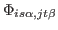, since the frequencies at wavevector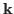are the eigenvalues of the dynamical matrix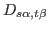, defined as: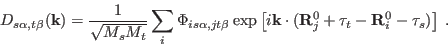(1)

where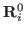is a vector of the lattice connecting different primitive cells and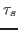is the position of the atom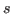in the primitive cell. If we have the complete force-constant matrix, thenand hence the frequencies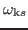can be obtained at any, so that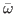can be computed to any required precision. In principle, the elements ofare non-zero for arbitrarily large separations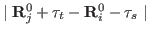, but in practice they decay rapidly with separation, so that a key issue in achieving our target precision is the cut-off distance beyond which the elements can be neglected.

Dario Alfe` 2012-02-20